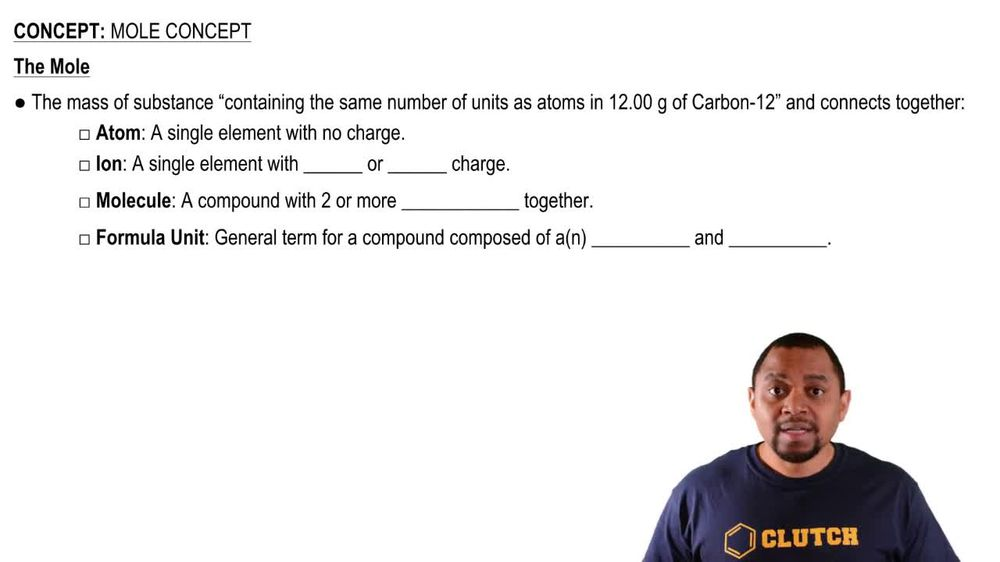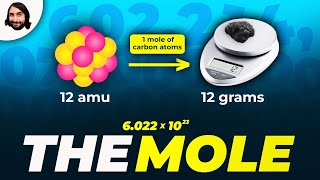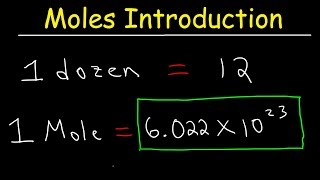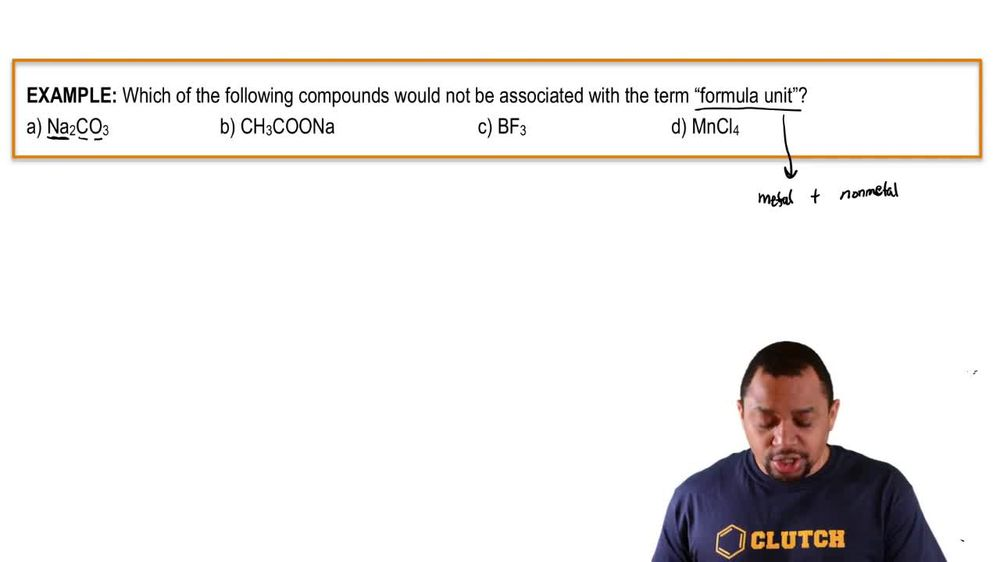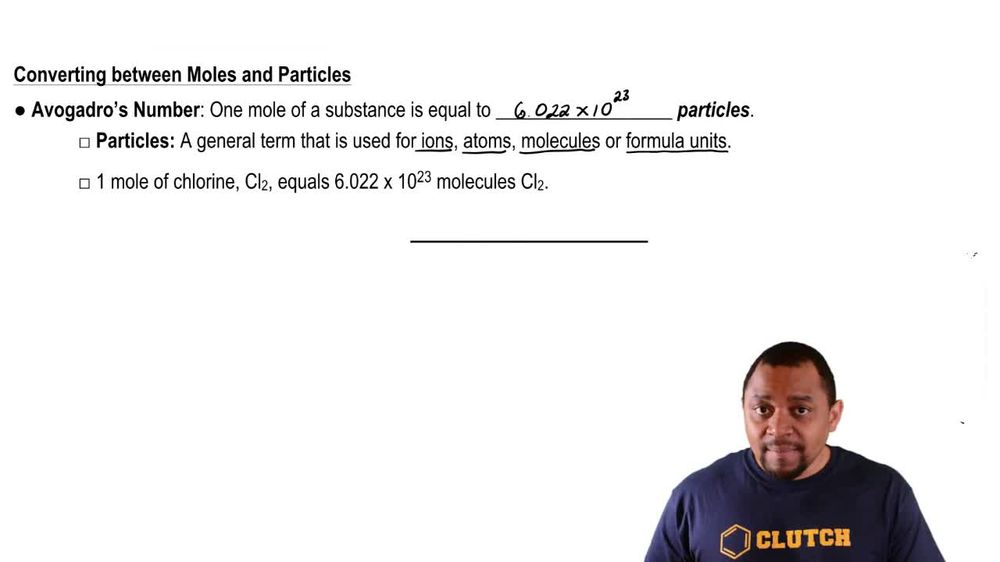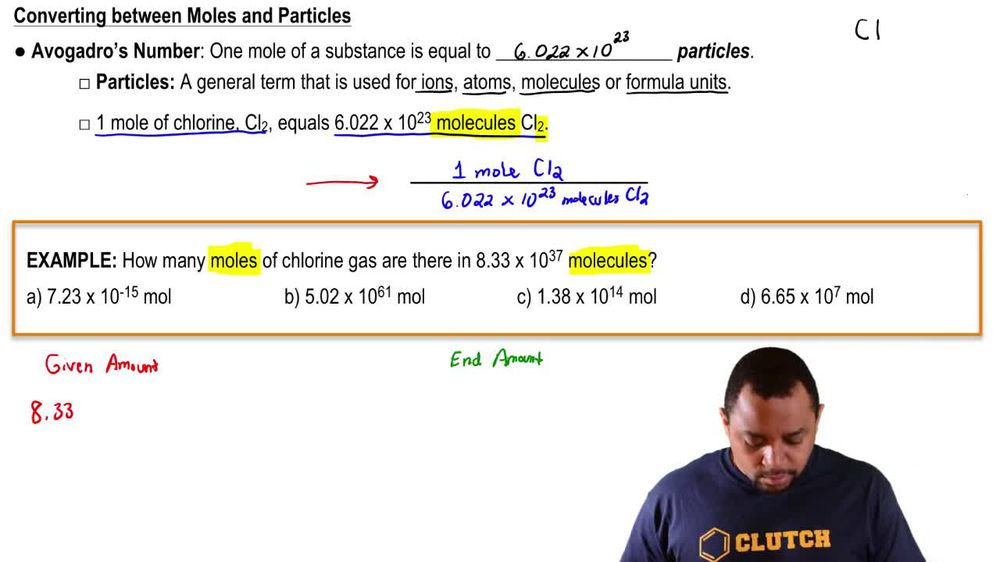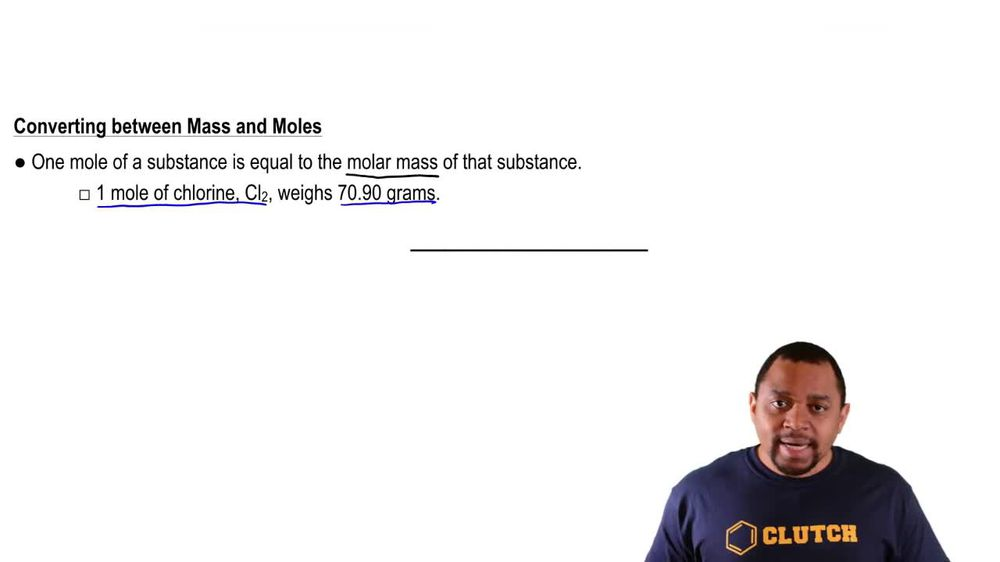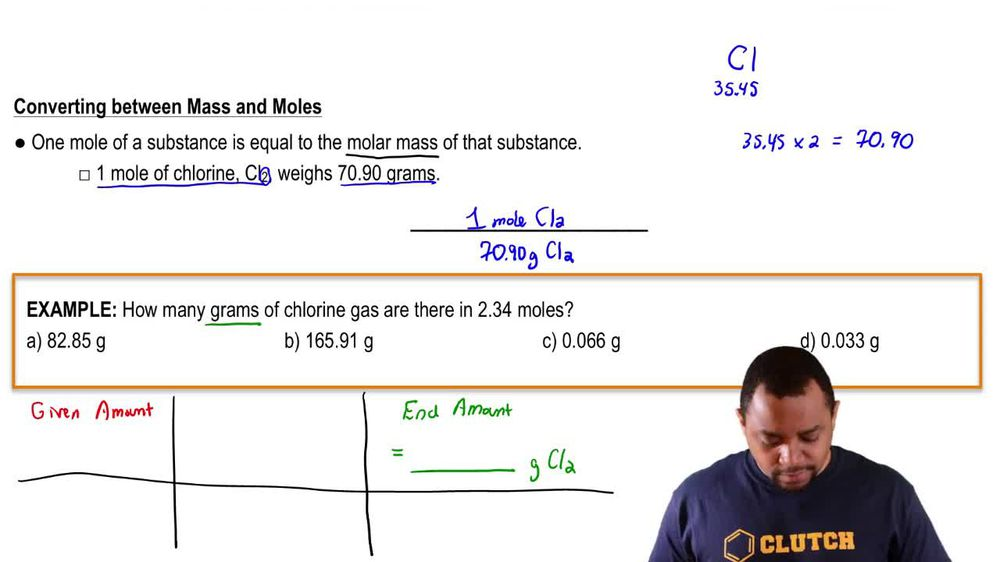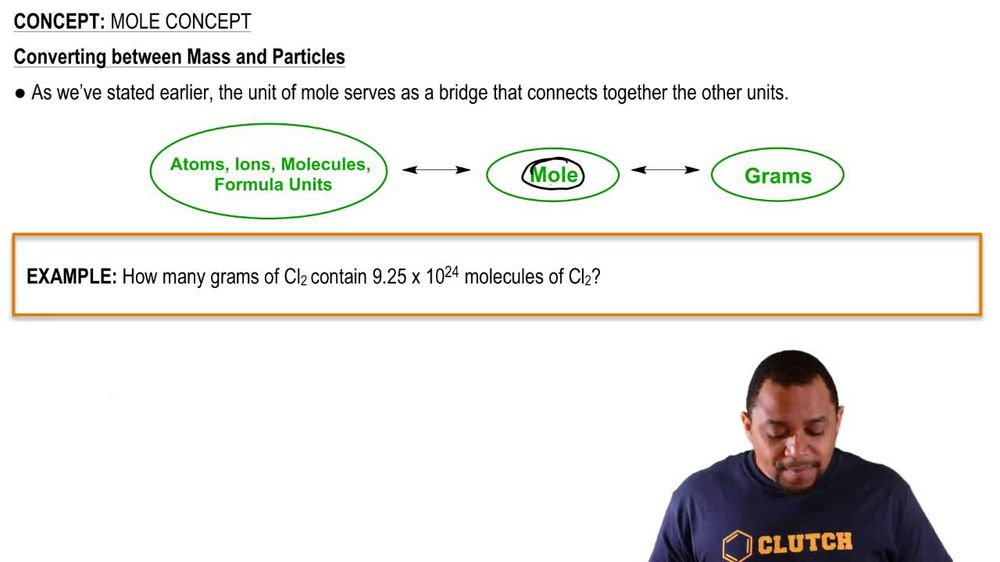Start typing, then use the up and down arrows to select an option from the list.
1. 2. Atoms & Elements2. Mole Concept
Problem

# (a) What is the mass, in grams, of 2.50 * 10-3 mol of ammonium phosphate?

Relevant Solution2m
Play a video:
Hi everyone here, we have a question asking us to calculate the mass and grams of a sample containing 5.69 times 10 to the negative fifth moles of calcium carbonate. So we have calcium With a Plus two charge. And that is because it is in the second group of the periodic table. And then we have carbonate Which has a -2 charge. So these are going to cancel out and give us calcium carbonate. Now we need to add our molar mass of calcium carbonate. So we have one calcium. And if we look on the periodic table has a molar mass of 40.08, We have one carbon With the molar mass of 12.01 And we have three oxygen With a molar mass of 16. And if we add this together, we get 100.9 g per mole. So we're going to take our 5.6- Times 10 to the negative 5th moles And multiply by 100 .9 g per mole. And our moles are going to cancel out. And that is going to give us 0.00569. Now, let's put that into scientific notation. So our six here, if we look to the right of it, we have a nine. So that's going to be rounded up to a seven. And we want to move our decimal place 1, 2, 3 places to the right. So we're gonna get 5. times 10 to the -3 g. And that is our final answer, thank you for watching. Bye